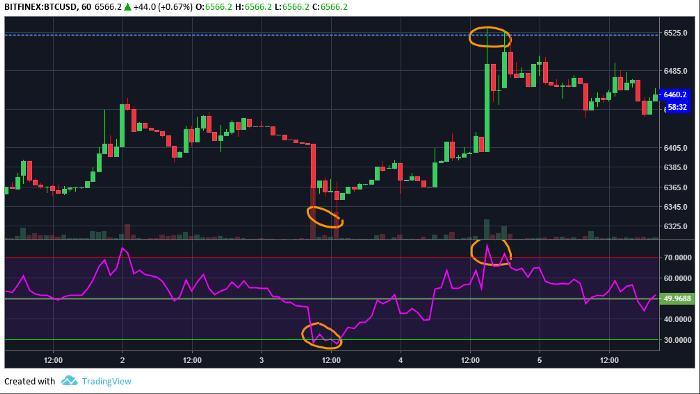初学者在数字货币量化交易中的6种简单策略及实践

Author: , Created: 2019-07-18 16:21:42, Updated: 2019-07-20 21:22:08

初学者的简单交易策略

• 长期持有（HODL方法）策略

• 日内交易策略

• 剥头皮交易策略

• 摇摆交易策略

• RSI交易策略

• 规避“大资金做庄的交易标的”（多为骗局）策略长期持有（HODL方法）策略

每日市价定投策略

function main() {
Log(exchange.GetAccount());

//最近一次投资的日期
var lastInvestDate = '';

while (true) {
//每次轮询，间隔时间为60秒
Sleep(60 * 1000);

//如果当前日期和最近一次投资日期相同，说明当天已经投过了，跳过
var now = new Date();
var date = now.toISOString().slice(0,10);
if (date == lastInvestDate) {
continue;
}

lastInvestDate = date;
Log("日期: " + date);

}
}

def onTick():

exchange_count = len(exchanges)
for i in range(exchange_count):
account = exchanges[i].GetAccount()

marketName = exchanges[i].GetName()
depth = exchanges[i].GetDepth()
Log("Market ",marketName,exchanges[i].GetCurrency(),"Account Balance [",account["Balance"],"] Stocks[",account["Stocks"],"]")
if account and depth and account["Balance"] > accountLimitMoney :
if bidPrice <  maxBidPrice :
amount = orderAmount
if amount <= account["Balance"]:
else:
Log("Account Balance is less than bid Amount")
else:
Log("Bid Price >= maxBidPrice, not process")
else:
Log("Account Balance <= accountLimitMoney")
def main() :
while 1:

onTick()
time.sleep(orderTimeInterval)日内交易策略

DIRECTION:=0;         // 方向控制
// Direction control

VAR2:=(HIGH+LOW+CLOSE)/3;
VAR3^^MA(VAR2,PARAM1);
VAR4^^EMA(VAR3,PARAM2);
BOOL1:=CLOSE>REF(C,1) AND HIGH>REF(HIGH,1) AND CLOSE>OPEN;
BOOL2:=CLOSE<REF(C,1) AND LOW<REF(LOW,1) AND CLOSE<OPEN;

BUYPK:=BARPOS>PARAM1 AND CLOSE>VAR3 AND BOOL1 AND VAR3>VAR4;
SELLPK:=BARPOS>PARAM1 AND CLOSE<VAR3 AND BOOL2 AND VAR3<VAR4;

SELLJ:=CLOSE<SKPRICE AND SELLPK;
SELLS:=CLOSE<BKPRICE*(1-PARAM3*0.01);

SKVOL=0 AND SELLPK AND DIRECTION<=0,SPK;
SKVOL>0 AND SELLJ,SK;
SELLS,SP;
AUTOFILTER;剥头皮交易策略摇摆交易策略

RSI或MACD等指标在使用较长时间范围时非常有用。他们的图表模式也可以用于摇摆交易，并且可以提供关于价格何时进入或退出的大量信息。

//MACD
MACDVALUE:=EMA(CLOSE,FASTLENGTH)-EMA(CLOSE,SLOWLENGTH);
AVGMACD:=EMA(MACDVALUE,MACDLENGTH);
MACDDIFF:MACDVALUE-AVGMACD;

//MA1、MA2
DMA1^^MA(C,L1);
DMA2^^MA(C,L2);

BUYCONDITION:=MACDVALUE>0 && DMA1>DMA2 && MACDDIFF>0 && C>DMA1 && REF(C,1)>REF(DMA1,1);
SELLCONDITION:=MACDVALUE<0 && DMA1<DMA2 && MACDDIFF<0 && C<DMA1 && REF(C,1)<REF(DMA1,1);

//开仓条件
SKVOL=0 AND SELLCONDITION,SK;

//离场条件
BKVOL>0 AND (REF(MACDVALUE,1)<0 OR REF(DMA1,1)<REF(DMA2,1)),SP;
SKVOL>0 AND (REF(MACDVALUE,1)>0 OR REF(DMA1,1)>REF(DMA2,1)),BP;

// 启动止损
SKVOL>0 AND HIGH>=卖出开仓价*(1+STOPLOSS*0.01),BP;
BKVOL>0 AND LOW<=买入开仓价*(1-STOPLOSS*0.01),SP;
AUTOFILTER;RSI交易策略

RSI是一个简单的动量指标，用于衡量近期价格走势的速度和变化，以帮助识别超买和超卖市场。More